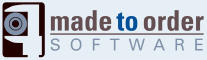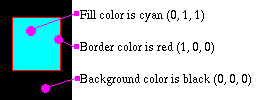# Appendix A — The geometry in SWF — Edges

Edges are used to define a shape vector based and also coordinates where images need to be drawn. The edges are always coordinates from where ever your last point was to where ever you want the next point to be (a little like a turtle in LOGO).

For vector based shapes to be placed anywhere in the screen and easily transformed with matrices, you should always create them centered properly (i.e. the center of the shape should be placed wherever you think it is the most appropriate in order to enable easy rotations - i.e. in a circle, the center of the circle should be selected and in a square the center of that square).

The fill styles and line styles can all be used together. The line style is fairly easy to understand. There is a width in TWIPS and a color. When a filled shape is being drawn using a line style it is used to draw the borders of the shape. There can be one or two fill styles. They both are drawn one after another wherever an area has to be filled. The filling scheme is a very simple even-odd scheme (i.e. don't draw till first line being crossed, then draw till next line, then don't draw till next line, etc.)Fig 1. Edges

The edges are defined either as a straight line record (a set of (x, y) coordinates) or a curve record (two sets of (x, y) coordinates). These coordinates are not absolute. Instead these are added to the previous coordinates. This usually enables for much better compression since these numbers are always very small.

The encoding even enables the definition of straight lines with the use of only the x or y offset for straight lines. Thus, if you create a square, with coordinates (-10,-10) and (+10,+10) you would define it as follow in SWF:Move to (-10, -10) [a setup edge] Draw to +20 by x Draw to +20 by y Draw to -20 by x Draw to -20 by y End Fig 2. Edges

The curves are simple B-splines defined by only three points (see Edges - Fig 3.):

• the starting point defined as the current position;
• the edge control point; it is the point which has an effect on the shape of the curve;
• and the anchor point which is the ending point of the curve;

Curves perfectly go through the starting and ending points which can therefore be perfectly continued by a straight line or another curve.Fig 3. Edges

A curve is defined as six curve segments Q3 to Q8. These are defined by duplicating the starting and ending points four times each giving a set of points similar to [A, A, A, A, B, C, C, C, C]. Edges - Fig. 4 shows the computation used to define each segment (i varying from 3 to 8). The parameter u can be given values from 0 to 1. The number of values will depend on the precision you need to draw the resulting curve.Fig 4. Edges

Though the math can be simplified in the case of SWF (since we only have three points), the following shows you a simple C code that can be used to draw such a curve (this is a cubic spline rendering.)

```struct point {
double		x;
double		y;
};

const double	Bmatrix = {
{ -1,  3, -3, 1 },
{  3, -6,  3, 0 },
{ -3,  0,  3, 0 },
{  1,  4,  1, 0 }
};

void compute_point(double u, const struct point *input_points, struct point *result_point)
{
double		Umatrix, Pmatrix, Imatrix, Rmatrix;

/* compute the U factors */
Umatrix = u * u * u;
Umatrix = u * u;
Umatrix = u;
Umatrix = 1;

/* because there is no Z it is set to zero */
Pmatrix = input_points.x;
Pmatrix = input_points.y;
Pmatrix = 0;
Pmatrix = 1;
Pmatrix = input_points.x;
Pmatrix = input_points.y;
Pmatrix = 0;
Pmatrix = 1;
Pmatrix = input_points.x;
Pmatrix = input_points.y;
Pmatrix = 0;
Pmatrix = 1;
Pmatrix = input_points.x;
Pmatrix = input_points.y;
Pmatrix = 0;
Pmatrix = 1;

/* compute I = UB */
Imatrix =    Umatrix * Bmatrix +
Umatrix * Bmatrix +
Umatrix * Bmatrix +
Umatrix * Bmatrix;

Imatrix =    Umatrix * Bmatrix +
Umatrix * Bmatrix +
Umatrix * Bmatrix +
Umatrix * Bmatrix;

Imatrix =    Umatrix * Bmatrix +
Umatrix * Bmatrix +
Umatrix * Bmatrix +
Umatrix * Bmatrix;

Imatrix =    Umatrix * Bmatrix +
Umatrix * Bmatrix +
Umatrix * Bmatrix +
Umatrix * Bmatrix;

/* I = I x 1/6 */
Imatrix /= 6.0;
Imatrix /= 6.0;
Imatrix /= 6.0;
Imatrix /= 6.0;

/* R = IP */
Rmatrix =    Imatrix * Pmatrix +
Imatrix * Pmatrix +
Imatrix * Pmatrix +
Imatrix * Pmatrix;

Rmatrix =    Imatrix * Pmatrix +
Imatrix * Pmatrix +
Imatrix * Pmatrix +
Imatrix * Pmatrix;

Rmatrix =    Imatrix * Pmatrix +
Imatrix * Pmatrix +
Imatrix * Pmatrix +
Imatrix * Pmatrix;

Rmatrix =    Imatrix * Pmatrix +
Imatrix * Pmatrix +
Imatrix * Pmatrix +
Imatrix * Pmatrix;

/* copy the result in user supplied result point */
result_point.x = Rmatrix;
result_point.y = Rmatrix;
}

void compute_curve(long repeat, const struct point *input_points, struct point *result_points)
{
/* we assume that the input_points are the three points of interest	   (namely: start, control and end) */
/* we assume that the result_points is a large enough array to receive	   the resulting points (depends on the repeat parameter) */
struct point		*points;
int			p, i;
double			u;

/* transform so compute_point() can easilly be used */
points = input_points;
points = input_points;
points = input_points;
points = input_points;
points = input_points;
points = input_points;
points = input_points;
points = input_points;
points = input_points;

/* we could have a way to define the very first and last points without	   calling the sub-function since these are equal to the input_points and	   input_points respectively */
for(p = 0, i = 0; i <= 5; i++) {
for(u = 0.0; u < 1.0; u += 1.0 / repeat, p++) {
compute_point(u, points + i, result_points + p);
}
}
}
```

Any good C programmer will see many possible simplifications in the `compute_point` code. The two main simplifications are the `Rmatrix` and `Rmatrix` which are not required (and therefore don't need to be computed). The division by 6 could be applied to the B matrix. `Umatrix` being 1.0, it could be ignored in the computations. etc.

With 3 points, you can also use the following code that is a quadratic spline computation with only (x, y) coordinates.

```struct point {
float x;
float y;
};

point cp;
point *rp;

... // initialize the 3 points of an SWF curve

int LOD = 10; // number of segments per spline
for(int i = 0; i < LOD; i++, rp++) {
float t = (float) i / (float) LOD;
float it = 1.0f - t;

// calculate blending factors
float b0 = it * it;
float b1 = 2 * it * t;
float b2 = t * t;

// calculate curve point
rp->x = b0 * cp.x + b1 * cp.x + b2 * cp.x;
rp->y = b0 * cp.y + b1 * cp.y + b2 * cp.y;
}
```